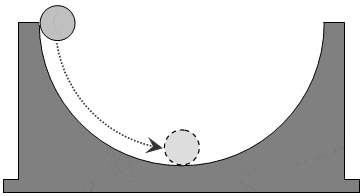# Rotational Kinetic Energy of a sphere

## Homework Statement

A small sphere, with radius 1.6 cm and mass 5.2 kg, rolls without slipping on the inside of a large fixed hemispherical bowl with radius 0.82 m and a vertical axis of symmetry. It starts at the top from rest. What is the kinetic energy of the sphere at the bottom?
What fraction of its kinetic energy at the bottom (in percent) is associated with rotation about an axis through its center of mass?## Homework Equations

KE = 1/2 mv2
KErot = 1/2Iw2
Vf2 = Vi2 + 2a(yf-yi)

## The Attempt at a Solution

I found the answer to the first question. My final equation was KE = mg(r2 - 0.5r1). r1 being the ball and r2 is the ramp.
My attempt at the second question was to find the KE and then subtract that from the total KE. When I came up with an equation for KE it was the same exact thing as the equation for my total KE, so I couldn't really subtract them to find the KErot.
I knew that KE = 1/2 mv2 so I used Vf2 = Vi2 + 2a(yf-yi) to find V2 and got that V2 = 2g(r1-0.5r2). Plugging this into the KE equation gives me the same equation [KE= mg(r[SUB]2[/SUB] - 0.5r1)]

$$\omega=\frac{v}{r}$$